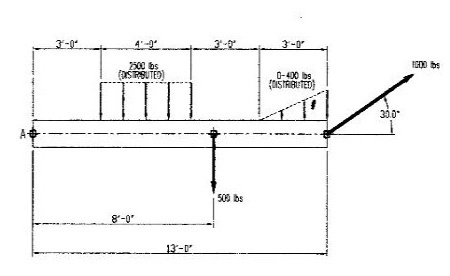# Determine the resultant and the location of the resultant for the forces and distributed loads...

## Question:

Determine the resultant and the location of the resultant for the forces and distributed loads shown in figure?## Magnitude and Location of Resultant Force on a Beam:

The applied loads on a beam can be simplified into a single force which is called resultant force. To do this, the sum of all x and y components of the loads are obtained. From there, the magnitude of the resultant force is calculated using the Pythagorean theorem. The angle of the resultant force is also needed to be reported. This is obtained using trigonometric identities. After the magnitude and orientation of the resultant force are obtained, the location where it acts on the beam should also be calculated. This is done by summing the individual moments of the forces and equating to the moment of the resultant force, where the only unknown is the distance of the resultant force to the reference point.

Become a Study.com member to unlock this answer! Create your account

For the forces along the y-direction, the total load is calculated below.

{eq}\downarrow F_y =...Resultant Force: Definition & Formula

from

Chapter 18 / Lesson 5
42K

In lots of situations, more than one force may act on an object. In this lesson, learn how to find the resultant force of two forces on a single object and how to use this to determine how the object will move.